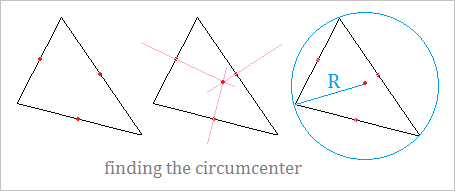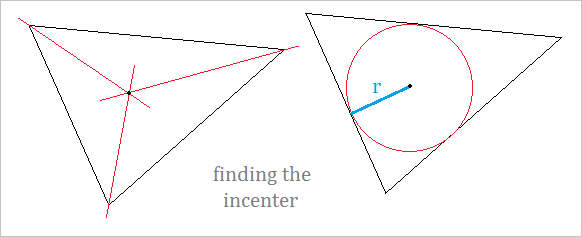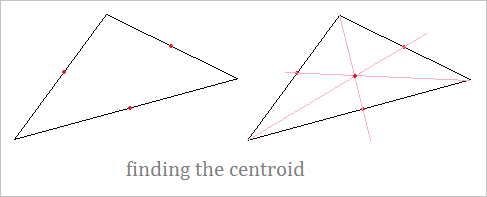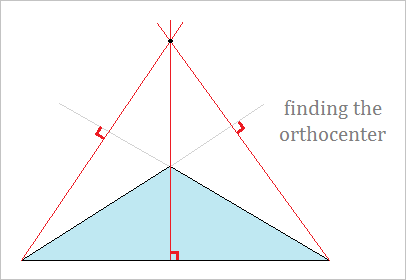# Triangular Center Points

Triangle Center Calculator
Enter three Cartesian coordinates below:

( , )
( , )
( , )

Area =
Circumcenter =
Incenter =
Orthocenter =
Centroid =

There are many ways to define the "center" of a two-dimensional shape. In the case of triangles, there are four fundamental kinds of central points. Depending on the shape of the triangle, some (or all) of the points may coincide.

The Circumcenter and Circumradius of a triangle are the center and radius of a circle that circumscribes the triangle.

The Incenter and Inradius of a triangle are the center and radius of a circle that can be inscribed within the triangle.

The Centroid of a triangle is its center of mass assuming uniform density.

The Orthocenter of a triangle is the intersection of the three altitudes.

Each center can be figured from the coordinates of the triangle's vertices or with a compass and straightedge. Interestingly, the circumcenter, centroid, and orthocenter always lie on the same line, called the Euler line. In isosceles, the incenter also lies on this line.

The triangle calculator on the left will compute the values when you input the Cartesian coordinates of the vertices.

### How to Find the Circumcenter

Determine the midpoints of each side of the triangle. Then, at each midpoint, draw a line perpendicular to the side of the triangle. Where the three lines intersect is the circumcenter. In obtuse triangles, the circumcenter is outside the triangle. In right triangles, the circumcenter is precisely the midpoint of the hypotenuse.If the sides of the triangle are a, b, c, and the area is E, then the radius (R) of the outer circle is given by the formula

R = abc/(4E)

### How to Find the Incenter

Bisect each angle of the triangle. Where the three angle bisectors meet is the incenter of the triangle.The inradius r is given by the formula

r = 2E/(a+b+c)

### How to Find the Centroid

A median is a line segment that connects a vertex of a triangle to the midpoint of the opposite side. Where the three medians meet is the centroid. If you know the coordinates of the vertices, you can calculate the centroid analytically. The x-coordinate of the centroid is the average of the x-coordinates of the vertices; the y-coordinate of the centroid is the average of the y-coordinates of the vertices.Besides being the barycenter of the three vertices, the centroid has other interesting properties. Drawing the three medians cuts the triangle into six smaller triangles of equal area. Furthermore, the centroid cuts each median into two segments, the longer segment being twice as long as the smaller segment.

### How to Find the Orthocenter

An altitude is a line that passes through a triangle's vertex perpendicular to the opposite side. When you draw the three altitudes of a triangle, they meet at a point called the orthocenter. In a right triangle, the orthocenter coincides with the right-angled vertex. In an obtuse triangle, the orthocenter is outside of the triangle.